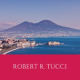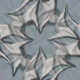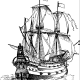# Quantum gravity from quantum error-correcting codes?

The lessons we learned from the Ryu-Takayanagi formula, the firewall paradox and the ER=EPR conjecture have convinced us that quantum information theory can become a powerful tool to sharpen our understanding of various problems in high-energy physics. But, many of the concepts utilized so far rely on entanglement entropy and its generalizations, quantities developed by Von Neumann more than 60 years ago. We live in the 21st century. Why don’t we use more modern concepts, such as the theory of quantum error-correcting codes?

In a recent paper with Daniel Harlow, Fernando Pastawski and John Preskill, we have proposed a toy model of the AdS/CFT correspondence based on quantum error-correcting codes. Fernando has already written how this research project started after a fateful visit by Daniel to Caltech and John’s remarkable prediction in 1999. In this post, I hope to write an introduction which may serve as a reader’s guide to our paper, explaining why I’m so fascinated by the beauty of the toy model.

This is certainly a challenging task because I need to make it accessible to everyone while explaining real physics behind the paper. My personal philosophy is that a toy model must be as simple as possible while capturing key properties of the system of interest. In this post, I will try to extract some key features of the AdS/CFT correspondence and construct a toy model which captures these features. This post may be a bit technical compared to other recent posts, but anyway, let me give it a try…

Bulk locality paradox and quantum error-correction

The AdS/CFT correspondence says that there is some kind of correspondence between quantum gravity on (d+1)-dimensional asymptotically-AdS space and d-dimensional conformal field theory on its boundary. But how are they related?

The AdS-Rindler reconstruction tells us how to “reconstruct” a bulk operator from boundary operators. Consider a bulk operator$\phi$ and a boundary region A on a hyperbolic space (in other words, a negatively-curved plane). On a fixed time-slice, the causal wedge of A is a bulk region enclosed by the geodesic line of A (a curve with a minimal length). The AdS-Rindler reconstruction says that$\phi$ can be represented by some integral of local boundary operators supported on A if and only if$\phi$ is contained inside the causal wedge of A. Of course, there are multiple regions A,B,C,… whose causal wedges contain$\phi$, and the reconstruction should work for any such region.

That a bulk operator in the causal wedge can be reconstructed by local boundary operators, however, leads to a rather perplexing paradox in the AdS/CFT correspondence. Consider a bulk operator$\phi$ at the center of a hyperbolic space, and split the boundary into three pieces, A, B, C. Then the geodesic line for the union of BC encloses the bulk operator, that is,$\phi$ is contained inside the causal wedge of BC. So,$\phi$ can be represented by local boundary operators supported on BC. But the same argument applies to AB and CA, implying that the bulk operator$\phi$ corresponds to local boundary operators which are supported inside AB, BC and CA simultaneously. It would seem then that the bulk operator$\phi$ must correspond to an identity operator times a complex phase. In fact, similar arguments apply to any bulk operators, and thus, all the bulk operators must correspond to identity operators on the boundary. Then, the AdS/CFT correspondence seems so boring…The bulk operator at the center is contained inside causal wedges of BC, AB, AC. Does this mean that the bulk operator corresponds to an identity operator on the boundary?

Almheiri, Dong and Harlow have recently proposed an intriguing way of reconciling this paradox with the AdS/CFT correspondence. They proposed that the AdS/CFT correspondence can be viewed as a quantum error-correcting code. Their idea is as follows. Instead of$\phi$ corresponding to a single boundary operator,$\phi$ may correspond to different operators in different regions, say$O_{AB}$,$O_{BC}$,$O_{CA}$ living in AB, BC, CA respectively. Even though$O_{AB}$,$O_{BC}$,$O_{CA}$ are different boundary operators, they may be equivalent inside a certain low energy subspace on the boundary.

This situation resembles the so-called quantum secret-sharing code. The quantum information at the center of the bulk cannot be accessed from any single party A, B or C because$\phi$ does not have representation on A, B, or C. It can be accessed only if multiple parties cooperate and perform joint measurements. It seems that a quantum secret is shared among three parties, and the AdS/CFT correspondence somehow realizes the three-party quantum secret-sharing code!

Entanglement wedge reconstruction?

Recently, causal wedge reconstruction has been further generalized to the notion of entanglement wedge reconstruction. Imagine we split the boundary into four pieces A,B,C,D such that A,C are larger than B,D. Then the geodesic lines for A and C do not form the geodesic line for the union of A and C because we can draw shorter arcs by connecting endpoints of A and C, which form the global geodesic line. The entanglement wedge of AC is a bulk region enclosed by this global geodesic line of AC. And the entanglement wedge reconstruction predicts that$\phi$ can be represented as an integral of local boundary operators on AC if and only if$\phi$ is inside the entanglement wedge of AC .

Building a minimal toy model; the five-qubit code

Okay, now let’s try to construct a toy model which admits causal and entanglement wedge reconstructions of bulk operators. Because I want a simple toy model, I take a rather bold assumption that the bulk consists of a single qubit while the boundary consists of five qubits, denoted by A, B, C, D, E.

What does causal wedge reconstruction teach us in this minimal setup of five and one qubits? First, we split the boundary system into two pieces, ABC and DE and observe that the bulk operator$\phi$ is contained inside the causal wedge of ABC. From the rotational symmetries, we know that the bulk operator$\phi$ must have representations on ABC, BCD, CDE, DEA, EAB. Next, we split the boundary system into four pieces, AB, C, D and E, and observe that the bulk operator$\phi$ is contained inside the entanglement wedge of AB and D. So, the bulk operator$\phi$ must have representations on ABD, BCE, CDA, DEB, EAC. In summary, we have the following:

• The bulk operator must have representations on R if and only if R contains three or more qubits.

This is the property I want my toy model to possess.

What kinds of physical systems have such a property? Luckily, we quantum information theorists know the answer; the five-qubit code. The five-qubit code, proposed here and here, has an ability to encode one logical qubit into five-qubit entangled states and corrects any single qubit error. We can view the five-qubit code as a quantum encoding isometry from one-qubit states to five-qubit states:$\alpha | 0 \rangle + \beta | 1 \rangle \rightarrow \alpha | \tilde{0} \rangle + \beta | \tilde{1} \rangle$

where$| \tilde{0} \rangle$ and$| \tilde{1} \rangle$ are the basis for a logical qubit. In quantum coding theory, logical Pauli operators$\bar{X}$ and$\bar{Z}$ are Pauli operators which act like Pauli X (bit flip) and Z (phase flip) on a logical qubit spanned by$| \tilde{0} \rangle$ and$| \tilde{1} \rangle$. In the five-qubit code, for any set of qubits R with volume 3, some representations of logical Pauli X and Z operators,$\bar{X}_{R}$ and$\bar{Z}_{R}$, can be found on R. While$\bar{X}_{R}$ and$\bar{X}_{R'}$ are different operators for$R \not= R'$, they act exactly in the same manner on the codeword subspace spanned by$| \tilde{0} \rangle$ and$| \tilde{1} \rangle$. This is exactly the property I was looking for.

Holographic quantum error-correcting codes

We just found possibly the smallest toy model of the AdS/CFT correspondence, the five-qubit code! The remaining task is to construct a larger model. For this goal, we view the encoding isometry of the five-qubit code as a six-leg tensor. The holographic quantum code is a network of such six-leg tensors covering a hyperbolic space where each tensor has one open leg. These open legs on the bulk are interpreted as logical input legs of a quantum error-correcting code while open legs on the boundary are identified as outputs where quantum information is encoded. Then the entire tensor network can be viewed as an encoding isometry.

The six-leg tensor has some nice properties. Imagine we inject some Pauli operator into one of six legs in the tensor. Then, for any given choice of three legs, there always exists a Pauli operator acting on them which counteracts the effect of the injection. An example is shown below:In other words, if an operator is injected from one tensor leg, one can “push” it into other three tensor legs.

Finally, let’s demonstrate causal wedge reconstruction of bulk logical operators. Pick an arbitrary open tensor leg in the bulk and inject some Pauli operator into it. We can “push” it into three tensor legs, which are then injected into neighboring tensors. By repeatedly pushing operators to the boundary in the network, we eventually have some representation of the operator living on a piece of boundary region A. And the bulk operator is contained inside the causal wedge of A. (Here, the length of the curve can be defined as the number of tensor legs cut by the curve). You can also push operators into the boundary by choosing different tensor legs which lead to different representations of a logical operator. You can even have a rather exotic representation which is supported non-locally over two disjoint pieces of the boundary, realizing entanglement wedge reconstruction.

What’s next?

This post is already pretty long and I need to wrap it up…

Shor’s quantum factoring algorithm is a revolutionary invention which opened a whole new research avenue of quantum information science. It is often forgotten, but the first quantum error-correcting code is another important invention by Peter Shor (and independently by Andrew Steane) which enabled a proof that the quantum computation can be performed fault-tolerantly. The theory of quantum error-correcting codes has found interesting applications in studies of condensed matter physics, such as topological phases of matter. Perhaps then, quantum coding theory will also find applications in high energy physics.

Indeed, many interesting open problems are awaiting us. Is entanglement wedge reconstruction a generic feature of tensor networks? How do we describe black holes by quantum error-correcting codes? Can we build a fast scrambler by tensor networks? Is entanglement a wormhole (or maybe a perfect tensor)? Can we resolve the firewall paradox by holographic quantum codes? Can the physics of quantum gravity be described by tensor networks? Or can the theory of quantum gravity provide us with novel constructions of quantum codes?

I feel that now is the time for quantum information scientists to jump into the research of black holes. We don’t know if we will be burned by a firewall or not … , but it is worth trying.

1. Whether entanglement wedge reconstruction is possible in the AdS/CFT correspondence or not still remains controversial. In the spirit of the Ryu-Takayanagi formula which relates entanglement entropy to the length of a global geodesic line, entanglement wedge reconstruction seems natural. But that a bulk operator can be reconstructed from boundary operators on two separate pieces A and C non-locally sounds rather exotic. In our paper, we constructed a toy model of tensor networks which allows both causal and entanglement wedge reconstruction in many cases. For details, see our paper.

This entry was posted in The expert's corner, Theoretical highlights by Beni Yoshida. Bookmark the permalink.I am a David and Ellen Lee postdoctoral scholar at Caltech, affiliated with the Institute for Quantum Information and Matter & the Walter-Burke Institute for Theoretical Physics.

## 17 thoughts on “Quantum gravity from quantum error-correcting codes?”

1.rrtucci on said:

So what is the classical probabilistic analogy of this, if there is one? And what is a perfect tensor, in simple terms?

2. Question  In what respects do the methods and insights of “Holographic quantum error-correcting codes …” (arXiv:1503.06237) apply to sonic black holes (a.k.a. acoustic black holes, analogue black holes, etc)?

A followup post on this topic would be welcomed by many (including me). For example, what is the acoustic analog of AdS/CFT correspondence? Is it simply the solution of the (linear) acoustic field equations for Neumann boundary conditions?

Especially welcome would be a tutorial walk-through of an (idealized) 1-d experiment acoustic black-hole experiment from beginning to end, accompanied by a dictionary: (1) thermal bath of phonons in a fluid [microwave radiation in space], then (2) startup of the flow [deformation of the metric], (3)  attainment of critical velocity [formation of an event horizon], (4)  singular cusp in local energy density [formation of a singularity], (5a)  transition from known acoustic physics to unknown atomic physics [transition from known geometric to unknown pre-geometric physics], (5b)  concomitant appeal to general thermodynamic constraints upon shock/detonation waves [concomitant appeal to general thermodynamic properties of firewalls].

In any event, thanks for this fine weblog, and for sharing this very interesting work!

• N.B.  In the world of sonic black holes, if we entangle a phonon-pair, and then send one of these photons into an acoustic firewall, and then slow the flow such that the shock-firewall cools back to normal fluid (with attendant emission of firewall energy as quasi-thermal phonons), then formally the starting entanglement is present in the shock-firewall emission (because shock-dynamics is formally unitary), yet computationally it is infeasible to extract (by measurement) that entanglement.

Broad question  (6) Supposing that we pullback Hilbert-space dynamics onto varietal subspaces (as is done in practice by large-scale quantum simulation codes [Jaguar:2013]), so as to simulate the dynamics with computational resources in P, then what elements of the above dynamical understanding survive (6a) exactly, versus (6b) approximately with exponentially small error, versus (6c) approximately with polynomially small error, versus (6d) not at all?).

Resolved for purposes of debate  Quantum firewalls and quantum simulation algorithms are comparably mysterious (albeit the latter are easier to observe) and it is reasonable to conceive that these two great mysteries are strongly entangled.


@article{Jaguar:2013, Author = {Bochevarov, Art D. and
Harder, Edward and Hughes, Thomas F. and Greenwood,
Jeremy R. and Braden, Dale A. and Philipp, Dean M. and
Rinaldo, David and Halls, Mathew D. and Zhang, Jing and
Friesner, Richard A.}, Journal = {International Journal
of Quantum Chemistry}, Number = {18}, Pages =
{2110--2142}, Title = {Jaguar: A high-performance quantum
chemistry software program with strengths in life and
materials sciences}, Volume = {113}, Year = {2013}}

• Twentieth century discussions regarding classical firewalls, that can be read as ancestral to 21st century discussions regarding quantum firewalls, are surveyed in two recent Bull. AMS articles: Denis Serre’s “Von Neumann’s comments about existence and uniqueness for the initial-boundary
value problem in gas dynamics” (2010), which is accompanied by a reprint of von Neumann’s comments “Discussion on the existence and uniqueness or multiplicity of solutions of the aerodynamical equation” (1949). The accompanying comments by Heisenberg, von Karman, and Burger retain considerable contemporary interest too (as Serre’s article discusses).

In particular, von Neumann’s comments tiptoed right up to the boundary of what was then-classified in regard to the theory of detonation (shock) waves. Indeed, Heisenberg had only recently been rehabilitated in regard to his research in service of the Nazis. Similarly, Soviet dynamicists were notably absent from this conference, plausibly because the Soviets were only ten days away from conducting their own first atomic test.

This helps us to appreciate a great merit of modern quantum firewall analyses (and many other aspects of quantum information science too): these analyses are not directly connected to issues of national security, state secrecy, or trade secrecy.

 @article{cite-key, Author = {von Neumann, J.}, Journal = {Bulletin of the American Mathematical Society}, Number = {1}, Pages = {145--154}, Title = {Discussion on the existence and uniqueness or multiplicity of solutions of the aerodynamical equation}, Volume = {47}, Year = {2010}} @article{cite-key, Author = {Denis Serre}, Journal = {Bulletin of the {AMS}}, Number = {1}, Pages = {139--144}, Title = {Von Neumann's comments about existence and uniqueness for the initial-boundary value problem in gas dynamics}, Volume = {47}, Year = {2010}} 

3.alex_v on said:

The paper mentioned in the post is also discussing three qutrits ECC. I wonder, if such short code may correct any single qutrit error? It is written about recovering after erasure – does it include all possible kinds of single qutrit errors from U(3)? And next, could the qutrit ECC be considered as an argument against Gil Kalai’s point about lack of analogue of classical repetition code?

4.Emi on said:

Reblogged this on Pathological Handwaving and commented:
I wonder if my abstract professor has seen this.